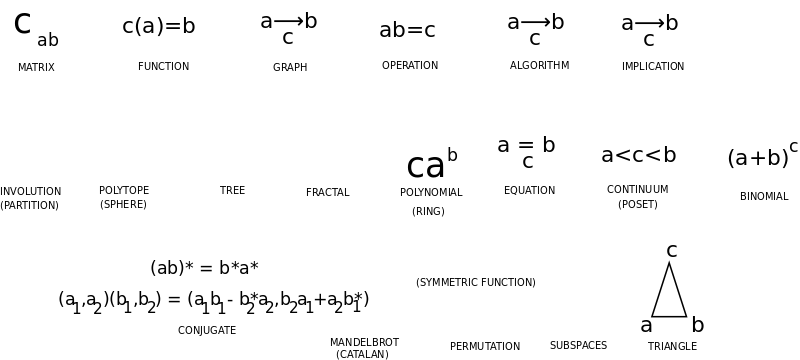• ms@ms.lt
• +370 607 27 665
• My work is in the Public Domain for all to share freely.

Introduction E9F5FC

Understandable FFFFFF

Questions FFFFC0

Notes EEEEEE

Software

See: Math Big Picture

Overview all of the concepts in math and how they arise.

• What is mathematical knowledge? How does it arise, develop and take the form of objects, concepts, theorems and endeavors?
• What are the roles of abstraction-generalization and concrete examples?
• Define the most basic concepts, especially the categories below.
• Organize the basic concepts. Show how they arise from the way we relate several variables and interpret duality.

On this page I am organizing a list of mathematical concepts that I wish to be able to account for. Sources of concepts include:

Basic ideas

• The most central concept in Math is duality, which is the basis for logic.
• The most fundamental symmetry is that of choice, as given by the ways we may interpret the binomial theorem, for it underlies the symmetry of algebraic expansions.Matematikos savokų pagrindas yra dvejybinis-trejybinis - operacijos jungia du narius trečiu nariu. Matricų elementai sieja du narius ir išgauna trečią. Kategorijų teorija panašiai. O geometrija lygiaverčiai sieja tris narius trikampiais, įvairiai suprastais. Tad tai paaiškintų geometrijos svarbą.

Mathematical concepts

Objects and their Symmetry

• combinatorial principles
• mathematical shapes
• polygons, polyhedra and polytopes
• regular polytopes - points on a circle or sphere, etc.
• partition topics
• factorial and binomial topics
• convexity topics

Binomial, Choice, Probability, Entropy

• Definite / deterministic (the) vs. Indefinite / Nondeterministic (a) - P vs. NP
• Axiom of Choice

Numbers Real, Complex, Quaternion

Functions

• mathematical functions
• special functions and eponyms
• transforms
• Fourier-related transforms
• polynomial topics
• exponential topics
• logarithm topics

Equations

• equations
• inequalities
• mathematical identities
• formulae involving pi

Subspaces

• curves
• surfaces
• algebraic surfaces
• manifolds
• examples in general topology

Operations

• small groups
• finite simple groups
• simple Lie groups
• Table of Lie groups
• complex reflection groups
• permutation topics

Algorithms

• algorithms
• complexity classes

Proofs

• axioms
• conjectures
• lemmas
• mathematical proofs
• theorems

Notes on concepts

• Function can be partial, whereas a permutation maps completely.
• Intrinsic ambiguity of propositions - every proposition is a general rule, which can be questioned or applied.

#### MathConcepts

Naujausi pakeitimai

 Puslapis paskutinį kartą pakeistas 2019 vasario 13 d., 14:34